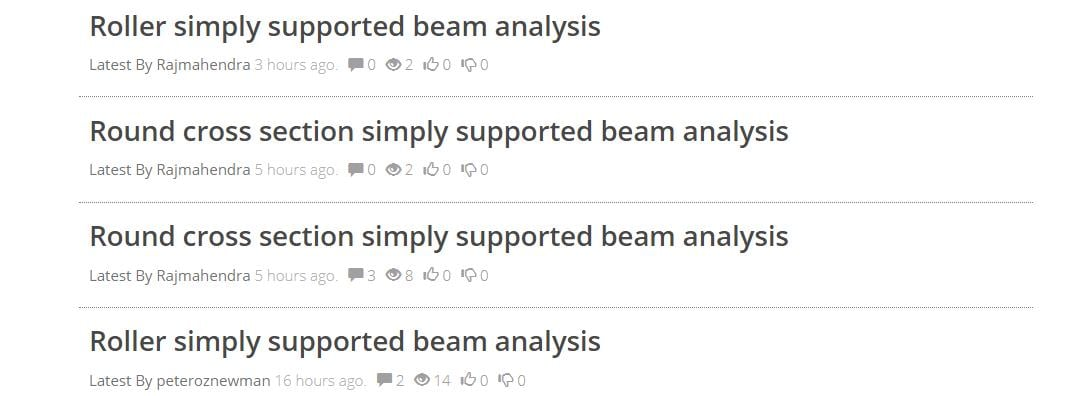## 3D Design

•aakib.vora
Subscriber
Hello My question is how to apply displacement and fixed suport in simply supported beam (round cross section)??
And also where to apply this force and displacement either on edge/ surface/ point?? And how it should be done in ansys aim
•peteroznewman
Subscriber

Hello Rajmahendra,

What is a "roller cross section"?  Please include an image in your reply and mention the version of AIM you are using.

When you say beam, I understand you mean a long straight object with a constant cross section.

ANSYS can analyze beams in two ways: using solid elements or using beam elements.
The choice of solid or beam elements dictates the kind of loads and supports that will be used, so choose that first.
A solid model will have a face at each end, and a face on the sides, which can be split to apply a "point load" in the center.
A beam model will have a vertex at each end and a line between them, which can be split to apply a "point load" in the center.
Nodes on the beam model each have six degrees of freedom: three translational and three rotational.

Let me define some terms that you have used to put them in terms of what ANSYS will do with those in the context of a beam element model.

• Fixed Support = Zero displacement and zero rotation about all six degrees of freedom.

• Simply Supported = Zero displacements but rotations are free.
A beam simply supported at two ends leaves the rotation about the line unsupported and will cause the solver to fail.

• Fixed Rotations are available to prevent rotation about selected coordinate directions, and can leave some rotations free.
Use that at one end of the beam to prevent the solver from failing. If you do simply supported and fix all three rotations on the same vertex, that is the same as a Fixed Support.

• Displacement support allows specification of individual displacement degrees of freedom.
For example, the textbook simply supported beam diagram where the far end of the beam is on a roller support can be achieved by a displacement support where the x-axis is Free, while y-axis is 0 and the z-axis is 0 (assuming the beam lies along the x-axis).

Regards,

Peter

•aakib.vora
Subscriber
Sir I mean that I am having a "round cross section beam" which is simply supported (i.e fixed support at one end and displacement at other end ) having force at the center ( at mid point ) on top surface of the round 3d beam.

So my question is that how to apply this constraints of fixed support and displacement (i.e either on surface or edge or point) for simply supported beam. And how to apply force at the "mid point" of the outer surface of round beam.

I'm using ansys aim 18.1
•aakib.vora
Subscriber
Sir I mean that I am having a "round cross section beam" which is simply supported (i.e fixed support at one end and displacement at other end ) having force at the center ( at mid point ) on top surface of the round 3d beam.

So my question is that how to apply this constraints of fixed support and displacement (i.e either on surface or edge or point) for simply supported beam. And how to apply force at the "mid point" of the outer surface of round beam.

I'm using ansys aim 18.1
•peteroznewman
Subscriber

Rajmahendra,

You have started four New Discussions, but they are all on the same topic.The concept of a Discussion is that multiple posts of questions and answers are grouped under one Discussion. When you start a New Discussion and you get a reply, the idea is you use Add Post to respond with more information or ask a related question. I believe this discussion is simply an answer to my post in this other discussion.

Please use the Add Post to reply to responses you get from your New Discussion.  Only use New Discussion when you are asking a question on an unrelated topic.

Also, please delete the duplicate post. That sometimes happens to me when the website freezes.

Maybe the Admins can combine these four Discussions into one?

To answer your question above about how to apply a force at the center of the 3D round beam, edit the geometry in SpaceClaim.

1. Create a Plane at one end of the beam.

2. Move the plane to the center of the beam

3. Split the face of the beam with the plane.

You will have an edge in Mechanical to apply the force.

I will have to get on my other computer to look at AIM to answer the question on applying supports.

Kind regards,

Peter

•aakib.vora
Subscriber
Ohk sir, but let me to know about it, I'm stuct at that only. That is where to apply the fixed support and displacement in simply supported beam either on edge or point or surface. Or any other option that I have to use to constrain the round simply supported beam with a point load at the top.
•peteroznewman
Subscriber

•aakib.vora
Subscriber
Sir thanks for the explanation But I'm still in a doubt, i.e as in the case of "square" cross section simply supported beam, we apply fixed support to the "edge" of rectangular beam and displacement to "edge" at the other end of the beam ( by setting the respective axis to free and constant).

Similarly, if we consider the case of simply supported beam with round cross section, where we have to apply the fixed support and displacement i.e either on edge or point of circular cross section.

Because, as shown by you sandeep sir if we apply the fixed support to the surface of round cross section it will lead to the case of cantiliver beam.

I'm using ansys aim 18.1
•peteroznewman
Subscriber

Rajmahendra,